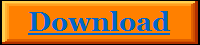Table of contest
Foreword
Chapter 1
1.1. The Ether
1.2. The structure of the quantized space

Chapter 2
2.1. The quantized magnetism
2.2. The quantized charge
2.3. The quantized mass
2.4. The quantized energy
2.6. The units invariance of the final formula

Chapter 3
3.1. Derivation of the electron mass
3.2. Derivation of the proton mass
3.4. Derivation of the proton radius
3.5. Derivation of the fine structure constant
3.6. Derivation of the acceleration due to gravity

Chapter 4
4.1. Derivation of the speed of light
4.2. The origin of time
4.3. The origin of spatial dimensions
4.4. The origin of pi

Chapter 5
5.1. The Planck time
5.3. The time factor in the natural constants

Chapter 6
6.1. The structure of the universe
6.2. The genesis of space
6.3. The expansion of the universe
6.4. The basic fundamental forces

Chapter 7
7.2. The Metalogic

The highlighted sections are excerpts in PDF.
Copyright © 2012 Halit Eroglu - Theory of everything  -       - Imprint - Contact - Sitemap
Theory  of  everything
This ebook is an English translation of the below work, which was published in Germany.
ISBN 978-3-8442-3885-3 "Die Weltformel - Die Urkraft des Universums" by Halit Eroglu
FREE eBook as PDF-File
Note:
The individual chapters and sections in this book are based on each. Since everything in the universe depends on one another, it is inevitable that one can understand much better the new world model and the final formula, after reading the whole book. Because, much more things will be more understandable in the overall context.